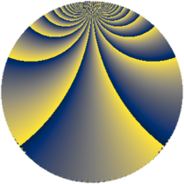# Properties

 Label 1344.4.cgLevel $1344$ Weight $4$ Character orbit 1344.cg Rep. character $\chi_{1344}(85,\cdot)$ Character field $\Q(\zeta_{16})$ Dimension $2304$ Sturm bound $1024$

# Related objects

## Defining parameters

 Level: $$N$$ $$=$$ $$1344 = 2^{6} \cdot 3 \cdot 7$$ Weight: $$k$$ $$=$$ $$4$$ Character orbit: $$[\chi]$$ $$=$$ 1344.cg (of order $$16$$ and degree $$8$$) Character conductor: $$\operatorname{cond}(\chi)$$ $$=$$ $$64$$ Character field: $$\Q(\zeta_{16})$$ Sturm bound: $$1024$$

## Dimensions

The following table gives the dimensions of various subspaces of $$M_{4}(1344, [\chi])$$.

Total New Old
Modular forms 6176 2304 3872
Cusp forms 6112 2304 3808
Eisenstein series 64 0 64

## Trace form

 $$2304q + O(q^{10})$$ $$2304q + 944q^{22} - 160q^{26} - 4960q^{32} - 4000q^{34} + 6560q^{40} + 2000q^{44} + 5952q^{51} - 864q^{54} + 1152q^{55} + 784q^{56} + 4896q^{60} + 11712q^{62} - 2016q^{63} - 1632q^{67} + 8256q^{68} + 2016q^{70} - 5264q^{74} + 8832q^{75} - 2976q^{76} + 11328q^{79} - 6240q^{88} - 17856q^{94} + O(q^{100})$$

## Decomposition of $$S_{4}^{\mathrm{new}}(1344, [\chi])$$ into newform subspaces

The newforms in this space have not yet been added to the LMFDB.

## Decomposition of $$S_{4}^{\mathrm{old}}(1344, [\chi])$$ into lower level spaces

$$S_{4}^{\mathrm{old}}(1344, [\chi]) \cong$$ $$S_{4}^{\mathrm{new}}(64, [\chi])$$$$^{\oplus 4}$$$$\oplus$$$$S_{4}^{\mathrm{new}}(192, [\chi])$$$$^{\oplus 2}$$$$\oplus$$$$S_{4}^{\mathrm{new}}(448, [\chi])$$$$^{\oplus 2}$$